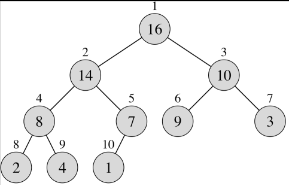## INTRODUCTION TO HEAP DATASTRUCTURE

Heap data structure is an array that can be seen as a complete binary tree .Heap in memory allocation is array thus elements are stored at contingious memory locations it can be represented as complete binary tree,each node of the tree corresponds to an element of the array .

The tree is completely filled on all levels except possibly the lowest,which is filled from the left up to a point.There are two kinds of binary heaps: max-heaps and min-heaps. In a max-heap, the max-heap property is that for every node i other than the root, the value of a node is at most the value of its parent. Thus, the largest element in a max-heap is stored at the root.

A min-heap is organized in the opposite way, each node is less than or equal to each of its children. Min-heaps are often used to implement priority queues.

Viewing a heap as a tree and a heap of n elements in based on a complete binary tree, its height is O (log n). Basic operations like insert, delete on heaps run in time at most proportional to the height of the tree and the take O (log n) time.

Why use a heap?

A heap can be thought of as a priority queue; the most important node will always be at the top, and when removed, its replacement will be the most important. This can be useful when coding algorithms that require certain things to processed in a complete order, but when you don't want to perform a full sort or need to know anything about the rest of the nodes. For instance, a well-known algorithm for finding the shortest distance between nodes in a graph, Dijkstra's Algorithm, can be optimized by using a priority queue.

Heaps can also be used to sort data. A heap sort is O(nlogn) efficiency, though it is not the fastest possible sorting algorithm.

Efficiency of a heap

Whenever you work with a heap, most of the time taken by the algorithm will be in upheaping and downheaping. As it happens, the maximum number of levels of a complete tree is log(n)+1, where n is the number of nodes in the tree. Because upheap or downheap moves an element from one level to another, the order of adding to or removing from a heap is O(logn), as you can make switches only log(n) times, or one less time than the number of levels in the tree .

Applications of HEAP Data Structures:

• to overcome the Worst-Case Complexity of Quick Sort algorithm from O(n^2) to O( nlog(n) ) in Heap Sort.

• find the smallest and largest element from a collection of array

• In the implementation of the Priority queue in graph algorithms like Dijkstra's algorithm Prim's algorithm and Huffman encoding

Happy Coding 😊

By Programmers Army

Contributed by: Parakh Pratap Singh